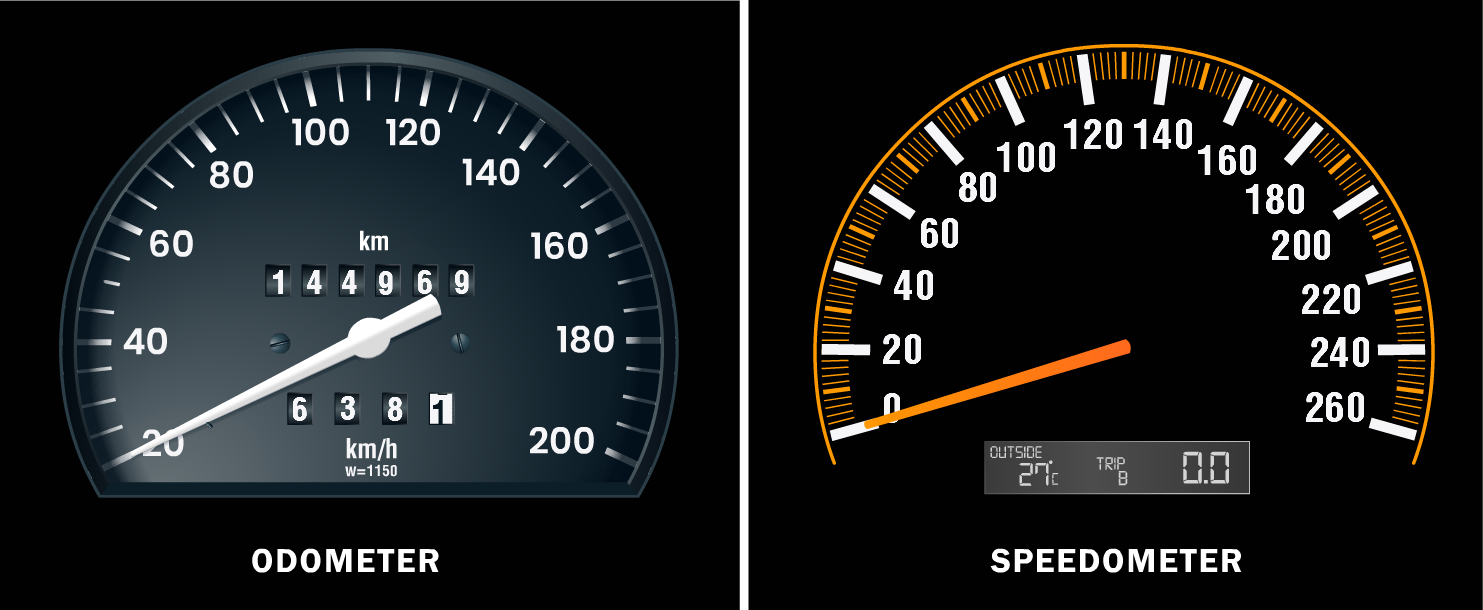Speed of Hoping on One Leg

# Our Objective

To find the speed of hopping on one leg.

# The Theory

## Speed and measuring speed

Galileo Galilei, an Italian physicist, is typically attributed with being the first to quantify speed by considering the distance travelled and the time required. Galileo defined speed as the distance covered per unit of time. The speed of an object, which is a scalar quantity in everyday usage and kinematics, is the magnitude of the change in that object's position over time or the magnitude of the change in that object's position per unit of time. The distance travelled by an object in a time interval is divided by the length of the interval to determine its average speed. You may have seen a metre placed on top of a motorcycle or scooter.

The dashboards of cars, buses, and other vehicles also have metres which take note of the km/h written in one corner of one of the metres. It is known as a speedometer.

The formula for how to find speed in physics is presented as:

Speed=distance/time

S=d/t

## Time

In physics, time is defined by its measurement that is time is what a clock reads but in classical, non-relativistic physics, it is called as scalar quantity and often denoted by the symbol ‘t.’ Time can be combined with other physical quantities to derive other concepts such as motion, kinetic energy, and time-dependent fields.# Learning Outcomes

• Students understand the concept of speed.
• Students understand the average speed.
• Students come to know about the concept of time used in physics.